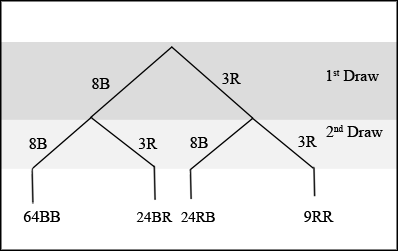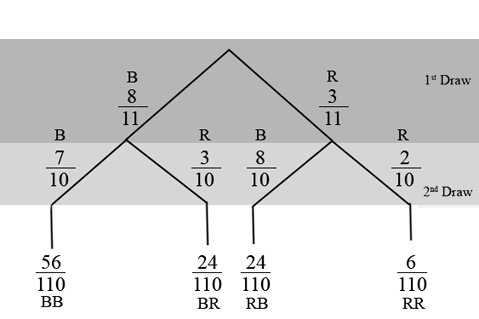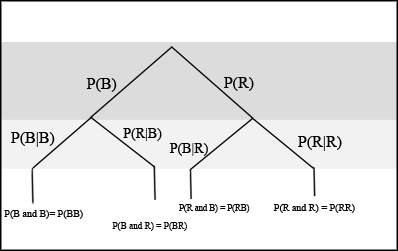# 3.6 Tree diagrams (optional)

 Page 1 / 3
This module introduces tree diagrams as a method for making some probability problems easier to solve. This module is included in the Elementary Statistics textbook/collection as an optional lesson.

A tree diagram is a special type of graph used to determine the outcomes of an experiment. It consists of "branches" that are labeled with either frequencies or probabilities. Tree diagrams can make some probability problems easier to visualize and solve. The following example illustrates how to use a tree diagram.

In an urn, there are 11 balls. Three balls are red ( $R$ ) and 8 balls are blue ( $B$ ). Draw two balls, one at a time, with replacement . "With replacement" means that you put the first ball back in the urn before you select the second ball. The tree diagram using frequencies that show all the possible outcomes follows.Total = 64 + 24 + 24 + 9 = 121

The first set of branches represents the first draw. The second set of branches represents the second draw. Each of the outcomes is distinct. In fact, we can list each red ball as $\mathrm{R1}$ , $\mathrm{R2}$ , and $\mathrm{R3}$ and each blue ball as $\mathrm{B1}$ , $\mathrm{B2}$ , $\mathrm{B3}$ , $\mathrm{B4}$ , $\mathrm{B5}$ , $\mathrm{B6}$ , $\mathrm{B7}$ , and $\mathrm{B8}$ . Then the 9 $\mathrm{RR}$ outcomes can be written as:

• $\mathrm{R1R1}$
• $\mathrm{R1R2}$
• $\mathrm{R1R3}$
• $\mathrm{R2R1}$
• $\mathrm{R2R2}$
• $\mathrm{R2R3}$
• $\mathrm{R3R1}$
• $\mathrm{R3R2}$
• $\mathrm{R3R3}$

The other outcomes are similar.

There are a total of 11 balls in the urn. Draw two balls, one at a time, and with replacement. There are outcomes, the size of the sample space .

List the 24 $\mathrm{BR}$ outcomes: $\mathrm{B1R1}$ , $\mathrm{B1R2}$ , $\mathrm{B1R3}$ , ...

• $\mathrm{B1R1}$
• $\mathrm{B1R2}$
• $\mathrm{B1R3}$
• $\mathrm{B2R1}$
• $\mathrm{B2R2}$
• $\mathrm{B2R3}$
• $\mathrm{B3R1}$
• $\mathrm{B3R2}$
• $\mathrm{B3R3}$
• $\mathrm{B4R1}$
• $\mathrm{B4R2}$
• $\mathrm{B4R3}$
• $\mathrm{B5R1}$
• $\mathrm{B5R2}$
• $\mathrm{B5R3}$
• $\mathrm{B6R1}$
• $\mathrm{B6R2}$
• $\mathrm{B6R3}$
• $\mathrm{B7R1}$
• $\mathrm{B7R2}$
• $\mathrm{B7R3}$
• $\mathrm{B8R1}$
• $\mathrm{B8R2}$
• $\mathrm{B8R3}$

Using the tree diagram, calculate $\text{P(RR)}$ .

$\text{P(RR)}=\frac{3}{11}\cdot \frac{3}{11}=\frac{9}{121}$

Using the tree diagram, calculate $\text{P(RB OR BR)}$ .

$\text{P(RB OR BR)}=\frac{3}{11}\cdot \frac{8}{11}+\frac{8}{11}\cdot \frac{3}{11}=\frac{48}{121}$

Using the tree diagram, calculate $\text{P(R on 1st draw AND B on 2nd draw)}$ .

$\text{P(R on 1st draw AND B on 2nd draw)}=\text{P(RB)}=\frac{3}{11}\cdot \frac{8}{11}=\frac{24}{121}$

Using the tree diagram, calculate $\text{P(R on 2nd draw given B on 1st draw)}$ .

$\text{P(R on 2nd draw given B on 1st draw)}=\text{P(R on 2nd | B on 1st)}=\frac{24}{88}=\frac{3}{11}$

This problem is a conditional. The sample space has been reduced to those outcomes that already have a blue on the first draw. There are possible outcomes (24 $\mathrm{BR}$ and 64 $\mathrm{BB}$ ). Twenty-four of the 88 possible outcomes are $\mathrm{BR}$ . $\frac{24}{88}=\frac{3}{11}$ .

Using the tree diagram, calculate $\text{P(BB)}$ .

Using the tree diagram, calculate $\text{P(B on the 2nd draw given R on the first draw)}$ .

There are outcomes that have $R$ on the first draw (9 $\mathrm{RR}$ and 24 $\mathrm{RB}$ ). The sample space is then . Twenty-four of the 33 outcomes have $B$ on the second draw. The probability is then $\frac{24}{33}$ .

An urn has 3 red marbles and 8 blue marbles in it. Draw two marbles, one at a time, this time without replacement from the urn. "Without replacement" means that you do not put the first ball back before you select the second ball. Below is a tree diagram. The branches are labeled with probabilities instead of frequencies. The numbers at the ends of the branches are calculated by multiplying the numbers on the two corresponding branches, for example, $\frac{3}{11}\cdot \frac{2}{10}=\frac{6}{110}$ .Total = 56 + 24 + 24 + 6 110 = 110 110 = 1
If you draw a red on the first draw from the 3 red possibilities, there are 2 red left to draw on the second draw. You do not put back or replace the first ball after you have drawn it. You draw without replacement , so that on the second draw there are 10 marbles left in the urn.

Calculate the following probabilities using the tree diagram.

$\text{P(RR)}$ =

$\text{P(RR)}=\frac{3}{11}\cdot \frac{2}{10}=\frac{6}{110}$

Fill in the blanks:

$\text{P(RB OR BR)}=\frac{3}{11}\cdot \frac{8}{10}+\text{(___)(___)}=\frac{48}{110}$

$\text{P(RB or BR)}=\frac{3}{11}\cdot \frac{8}{10}+$ $\left(\frac{8}{11}\right)\left(\frac{3}{10}\right)$ $=\frac{48}{110}$

$\text{P(R on 2d | B on 1st)}$ =

Fill in the blanks:

$\left(\frac{3}{11}\right)\left(\frac{8}{10}\right)$

$\text{P(BB)}$ =

=

There are outcomes that have $R$ on the first draw (6 $\mathrm{RR}$ and 24 $\mathrm{RB}$ ). The 6 and the 24 are frequencies. They are also the numerators of the fractions $\frac{6}{110}$ and $\frac{24}{110}$ . The sample space is no longer 110 but . Twenty-four of the 30 outcomes have $B$ on the second draw. The probability is then $\frac{24}{30}$ . Did you get this answer?

If we are using probabilities, we can label the tree in the following general way.• $\text{P(R|R)}$ here means $\text{P(R on 2nd | R on 1st)}$
• $\text{P(B|R)}$ here means $\text{P(B on 2nd | R on 1st)}$
• $\text{P(R|B)}$ here means $\text{P(R on 2nd | B on 1st)}$
• $\text{P(B|B)}$ here means $\text{P(B on 2nd | B on 1st)}$

#### Questions & Answers

Is there any normative that regulates the use of silver nanoparticles?
what king of growth are you checking .?
Renato
What fields keep nano created devices from performing or assimulating ? Magnetic fields ? Are do they assimilate ?
why we need to study biomolecules, molecular biology in nanotechnology?
?
Kyle
yes I'm doing my masters in nanotechnology, we are being studying all these domains as well..
why?
what school?
Kyle
biomolecules are e building blocks of every organics and inorganic materials.
Joe
anyone know any internet site where one can find nanotechnology papers?
research.net
kanaga
sciencedirect big data base
Ernesto
Introduction about quantum dots in nanotechnology
what does nano mean?
nano basically means 10^(-9). nanometer is a unit to measure length.
Bharti
do you think it's worthwhile in the long term to study the effects and possibilities of nanotechnology on viral treatment?
absolutely yes
Daniel
how to know photocatalytic properties of tio2 nanoparticles...what to do now
it is a goid question and i want to know the answer as well
Maciej
characteristics of micro business
Abigail
for teaching engĺish at school how nano technology help us
Anassong
Do somebody tell me a best nano engineering book for beginners?
there is no specific books for beginners but there is book called principle of nanotechnology
NANO
what is fullerene does it is used to make bukky balls
are you nano engineer ?
s.
fullerene is a bucky ball aka Carbon 60 molecule. It was name by the architect Fuller. He design the geodesic dome. it resembles a soccer ball.
Tarell
what is the actual application of fullerenes nowadays?
Damian
That is a great question Damian. best way to answer that question is to Google it. there are hundreds of applications for buck minister fullerenes, from medical to aerospace. you can also find plenty of research papers that will give you great detail on the potential applications of fullerenes.
Tarell
what is the Synthesis, properties,and applications of carbon nano chemistry
Mostly, they use nano carbon for electronics and for materials to be strengthened.
Virgil
is Bucky paper clear?
CYNTHIA
carbon nanotubes has various application in fuel cells membrane, current research on cancer drug,and in electronics MEMS and NEMS etc
NANO
so some one know about replacing silicon atom with phosphorous in semiconductors device?
Yeah, it is a pain to say the least. You basically have to heat the substarte up to around 1000 degrees celcius then pass phosphene gas over top of it, which is explosive and toxic by the way, under very low pressure.
Harper
Do you know which machine is used to that process?
s.
how to fabricate graphene ink ?
for screen printed electrodes ?
SUYASH
What is lattice structure?
of graphene you mean?
Ebrahim
or in general
Ebrahim
in general
s.
Graphene has a hexagonal structure
tahir
On having this app for quite a bit time, Haven't realised there's a chat room in it.
Cied
what is biological synthesis of nanoparticles
how did you get the value of 2000N.What calculations are needed to arrive at it
Privacy Information Security Software Version 1.1a
Good
Berger describes sociologists as concerned with
1 It is estimated that 30% of all drivers have some kind of medical aid in South Africa. What is the probability that in a sample of 10 drivers: 3.1.1 Exactly 4 will have a medical aid. (8) 3.1.2 At least 2 will have a medical aid. (8) 3.1.3 More than 9 will have a medical aid.ByByBy Nick Swain